本文所有代码均使用数组+结构体,不使用指针!

前言——BST是啥

BST 二叉搜索树是基于二叉树的一种树,一种特殊的二叉树。

1. 它的左子树要么为空,要么它(左子树)的所有节点均小于它的根节点。
2. 它的右子树要么为空,要么它(右子树)的所有节点均大于它的根节点。
3. 它的左、右子树也分别是二叉搜索树。

1. 有序
2. 插入、查找等算法高效

No.3——基本操作

BST的基本操作很多,一时半会也讲不过来,就从易到难的讲吧。

No.3-1——三种遍历方式

BST的遍历与二叉树和树一样,有三种:先序遍历、中序遍历、后续遍历。

1. 先序遍历:根→左→右 (DLR)
2. 中序遍历:左→根→右 (LDR) (结合定义,想一想,为什么中序遍历就是有序的？？)
3. 后续遍历:左→右→根 (LRD)

/*========遍历========*/
void bl(int how,int now)
{
if(how==1){        //先序遍历
cout<<a[now].data<<" ";
if(a[now].l!=-1)
bl(1,a[now].l);
if(a[now].r!=-1)
bl(1,a[now].r);
}
if(how==2){        //中序遍历
if(a[now].l!=-1)
bl(2,a[now].l);
cout<<a[now].data<<" ";
if(a[now].r!=-1)
bl(2,a[now].r);
}
if(how==3){        //后续遍历
if(a[now].l!=-1)
bl(3,a[now].l);
if(a[now].r!=-1)
bl(3,a[now].r);
cout<<a[now].data<<" ";
}
}

No.3-2——建树与插入

1. 第一个为根节点
2. 比根大,往右对比
3. 比根小,往左对比
4. 如果当前为空,插入成功。

/*========插入========*/
void into(int sum,int now,int tot)
{
if(sum<a[now].data)
if(a[now].l!=-1)
into(sum,a[now].l,tot);
else {
a[now].l=tot;
a[a[now].l].data=sum;
a[a[now].l].fa=now;
}
else if(sum>a[now].data)
if(a[now].r!=-1)
into(sum,a[now].r,tot);
else {
a[now].r=tot;
a[a[now].r].data=sum;
a[a[now].r].fa=now;
}
}
/*========构建========*/
void init()
{
cin>>n;
int x,tot;
for(tot=1;tot<=n;tot++){
scanf("%d",&x);
if(tot==1)
a[tot].data=x;
else
into(x,1,tot);
}
}

No.3-3——查找

/*========查找========*/
int find(int now,int sum)
{
cout<<now<<endl;
if(a[now].data==sum) return now;
if(sum<a[now].data)
if(a[now].l!=-1) return find(a[now].l,sum);
else return 0;
else if(sum>a[now].data)
if(a[now].r!=-1) return find(a[now].r,sum);
else return 0;
}
void init2()
{
cin>>n;
int i,x;
for(i=1;i<=n;i++){
cin>>x;
if(find(ROOT,x)) printf("Yes\n");
else printf("No\n");
}
}

No.3-4——求前驱后继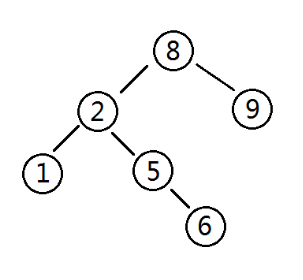/*========前驱========*/
int pred(int now)
{
if(a[now].r!=-1) return pred(a[now].r);
else return now;
}

No.3-5——删除

1. 没有孩子
2. 只有一个孩子
3. 有两个孩子

3-2. **删除根**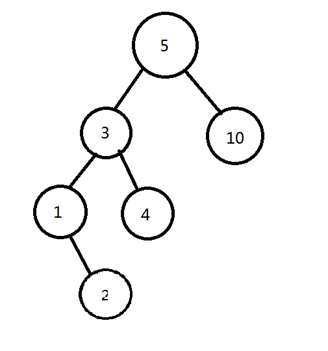这是一颗二叉搜索树,我们要删掉3。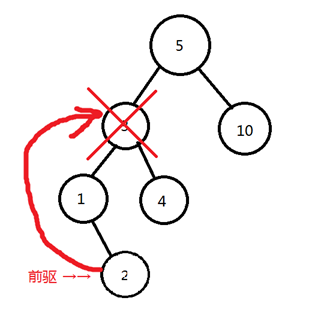找到前驱。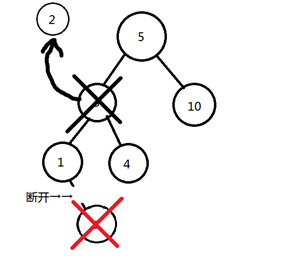替换。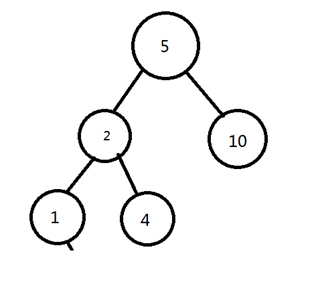最后变成这个样子。

No.4——写在最后

No.4-1——练习题

1. 模板题
2. [[HNOI2002]营业额统计](https://www.luogu.org/problemnew/show/P2234)

但,算法之路,才刚刚开始！

Last modification：July 21st, 2019 at 04:30 pm